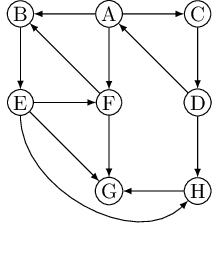# Glossary

## Algo: Directed acyclic graph

A cycle in a directed graph is a circular path $v_0 \rightarrow v_1 \rightarrow v_2 \rightarrow \dots \rightarrow v_k \rightarrow v_0$. The graph below has quite a few of them, for example, $B \rightarrow E \rightarrow F \rightarrow B$.A graph without cycles is acyclic. It turns out we can test for acyclicity in linear time, with a single depth-first search.

Property A directed graph has a cycle if and only if its depth-first search reveals a back edge.

Proof. One direction is quite easy: if $(u, v)$ is a back edge, then there is a cycle consisting of this edge together with the path from $v$ to $u$ in the search tree.

Conversely, if the graph has a cycle $v_0 \rightarrow v_1 \rightarrow v_2 \rightarrow \dots \rightarrow v_k \rightarrow v_0$, look at the first node on this cycle to be discovered (the node with the lowest pre number). Suppose it is $v_i$. All the other $v_j$ on the cycle are reachable from it and will therefore be its descendants in the search tree. In particular, the edge $v_{i−1} \rightarrow v_i$ (or $v_k \rightarrow v_0$ if $i = 0$) leads from a node to its ancestor and is thus by definition a back edge. ∎

Directed acyclic graphs (DAG), or dags for short, come up all the time. They are good for modeling relations like causalities, hierarchies, and temporal dependencies.